Data Structures and Algorithms with Object-Oriented Design Patterns in C#In this section we examine the asymptotic behavior of polynomials in n. In particular, we will see that as n gets large, the term involving the highest power of n will dominate all the others. Therefore, the asymptotic behavior is determined by that term.

Theorem  Consider a polynomial  in n of the formwhere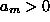. Then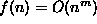.

extbfProof Each of the terms in the summation is of the form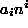. Since n is non-negative, a particular term will be negative only if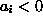. Hence, for each term in the summation,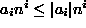. Recall too that we have stipulated that the coefficient of the largest power of n is positive, i.e.,.Note that for integers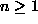,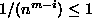for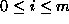. ThusFrom Equationwe see that we have found the constantsand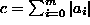, such that for all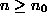,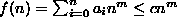. Thus,.

This property of the asymptotic behavior of polynomials is used extensively. In fact, whenever we have a function, which is a polynomial in n,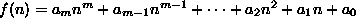we will immediately ``drop'' the less significant terms (i.e., terms involving powers of n which are less than m), as well as the leading coefficient,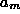, to write.Copyright © 2001 by Bruno R. Preiss, P.Eng. All rights reserved.